# Mathematical Operations with Binary, Hexadecimal and Octal Numbers

### 1 - Introduction

The use of numerical systems arose from the need of man to improve certain mathematical calculations as time goes.

Several factors demanded the study and application of mathematical operations using decimal numbers. Among these factors, technological advancement essentially from the first experiments in the use of electronics.

There are several numeric systems, but four of them stand out: decimal, binary, octal and hexadecimal.

The decimal system is used every day in countless ways and, no doubt, is the most important number system. He has ten digits with which can form any number through the law.

Non-decimal systems such as binary, octal and hexadecimal are critical in the areas of digital and computer techniques. Through these systems is possible to perform logical combinations and working with computer programming languages. This article will show the link between the logical circuits and these numbering systems.

### 2 - The Binary Numbering System

The binary numbering system is composed of only two digits:

Zero (0)

One (1)

To represent zero amount using the number 0; to represent a quantity, use the digit 1.

Assuming you need to represent the number two. Which digit you can use, if there is no number 2 in that system?

We have the following response. In the decimal system, we do not have the number ten and represent the amount of ten using the digit 1 followed by the digit 0. In this case, the number 1 means that we have a group of ten and the digit 0, no drive, which means ten.

In the binary system, do likewise. For you the amount of two, we use the digit 1 followed by the digit 0. The figure 1 means that there is a group of two elements and 0, a group of any unit, thus representing the number two.

The table below helps us to understand the differences between the decimal and binary system, using this rule. The number sequence displayed to the number nine.

 Decimal Binary 0 0 1 1 2 10 3 11 4 100 5 101 6 110 7 111 8 1000 9 1001

2.1 - System Conversion binary to Decimal System

To better understand the conversion we use a decimal number either, for example, 356. This number means:

3 x 100 + 5 x 10 + 6 x 1 = 356

Hundred ten unit

3 x 10 � + 5 x 10 � 6 x 10 + 10 = 356

We realize that the least significant digit (6) multiplies the unit (1), the second digit (5) multiplies the ten (10) and the most significant (3) multiplies the hundred (100). The sum of these results will represent the number.

In General, the formatting rule of a number is the sum of each digit multiplied by the corresponding base (in the example, the number ten) high for an index as the positioning of the digit in the number.

In another situation, we will use a binary number, for example, the number 101. We can conclude that it is equivalent to number 5 in the decimal system. Using the concept of a number, we will convert the number to the decimal system as follows:

1 0 1

1 x 2� + 0 x 2� + 1 x 2

1 x 4 + 0x2 + 1 x 1 = 5

The 101 number in base 2 is equal to 5 in base 10.

2.2  - System Conversion Decimal to Binary System

This is the inverse conversion when we saw earlier. The conversion of a decimal system number for the binary system.

Let's use as an example the number 67. The first step is to divide the 67 for 2. The first rest of this Division is 1. I.e. 2 x 33 + 1 = 67.

Figure 1 below shows how this process: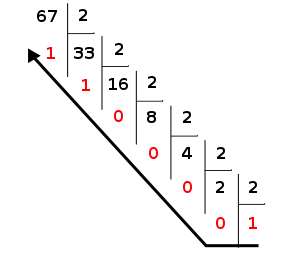Figure 1: process of Division of decimal number to binary.

Continuing, we split now 33 for 2. The second rest is 1. I.e. 16 x 2 + 1 = 33.

Dividing 16 for 2, results in the third rest 0 value. Soon, 8 x 2 + 0 = 16.

The following 8 Division by 2, presents the rest of 0 value too, and 4 x 2 + 0 = 8.

Continuing, we divided 4 by 2, that will result in the rest of value 0. Therefore, 2 x 2 + 0 = 4.

Finally, dividing 2 by 2, we have the last rest, with value 0 and the quotient of value 1.

This process is called successive divisions, which consists of making successive divisions for the base to be converted (in this case the 2) until the last possible quotient. The transformed number will consist of the latter quotient (most significant digit) and all the leftovers, in reverse order to the divisions.

In this way, we have in this example the binary number 1000011 2 base, equivalent to 67 in decimal.

3 - The Octal Numbering System

Currently, the octal system practically is little used in the area of Digital Electronics. This is a numeric system intermediate between binary and hexadecimal.

The octal numbering system is base 8 in which eight digits: 0, 1, 2, 3, 4, 5, 6 and 7.

The representation of the number eight is likewise of used for binary numbers and decimals. We put the digit 1 followed by the digit 0. So we have a group of eight added to any unit.

Using this concept, we set up a table with numbering sequence to represent other quantities. The table below shows the numbering of the octal system until the number sixteen.

 Decimal Octal 0 0 1 1 2 2 3 3 4 4 5 5 6 6 7 7 8 10 9 11 10 12 11 13 12 14 13 15 14 16 15 17 16 20

3.1 - Conversion of Octal System to decimalization

To convert an octal number to decimal, use the basic concept of a number, as already seen.

Let's look at an example to convert the number 122 in the base 8 to decimal.

 8� 8� 8 1 2 2

1 x 8� + 2 x 8� + 2 x 8 =

1 x 64 + 2 x 8 + 2 x 1 = 64 + 32 + 2 = 98

Soon, the number 122 in 8 base corresponds to 98 at the base 10.

3.2 - System Conversion Decimal to Octal system

The process is identical to the system conversion decimal to binary, only that in this case we use the Division by 8, because being the octal system, its base is equal to 8.

As an example, let's convert the number 217 on 10 base to octal system, shown in the figure below: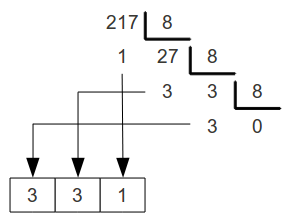In this case, the number 217 on 10 base amounts to 331 in base 8.

3.3 � Conversion of Octal to Binary System

Is a simpler conversion and we can use the following rule. We will use a any octal number, for example, 42 in base 8. The rule is to turn each digit directly in the corresponding binary digit, the default number of bits of the system, and for each octal three (2� = 8. Base of the octal system). In this case, we have:

4 2

100 010

Therefore, 42 in base 8 equals 100010 on base 2.

3.4 - System Conversion binary to Octal system

This conversion is exactly the reverse process to that used in converting octal to binary. Let's use as an example, the number in base 2 110010.

To make this number to octal, you must separate it into groups of 3 bits from the right:

110 010

To perform the conversion of each group of bits directly into the octal system, do the following:

110 010

6 2

The converted number consists of the Union of the figures obtained. It is concluded that the result of this conversion is in base 2 110010 equals 62 in base 8.

If there are cases of the latter group form incomplete, add leading zeros to complete it with 3 bits. To illustrate, let's convert the 1010 binary number to octal.

1 010

001 010 = 1010 = 12 in base 8.

1 2

4 - The Hexadecimal Numbering System

The system has 16 hexadecimal digits being your base equal to 16. The digits are: 0, 1, 2, 3, 4, 5, 6, 7, 8, 9, A, B, C, D, E and F.

Important to note that the letter A represents A numeral representing the number ten. The letter B represents the digit B representing the number eleven, and so on until the letter F representing the number fifteen.

To represent the number sixteen, we used the basic concept of a number, placing the digit 1 followed by the digit 0, representing a group of sixteen added to any unit.

The table below shows the sequence of hexadecimal numbering until the number twenty.

 Decimal Hexadecimal 0 0 1 1 2 2 3 3 4 4 5 5 6 6 7 7 8 8 9 9 10 A 11 B 12 C 13 D 14 E 15 F 16 10 17 11 18 12 19 13 20 14

Is a system widely used in the area of microprocessors and memory mapping in digital systems. Is a very important number system, when applied in software and hardware projects.

4.1 � System Conversion Hexadecimal to Decimal System

The conversion rule follows the same of other systems, but in this case the base is 16.

As an example, let's use the number in base 16 3F and convert it to decimal:

 16� 16 3 (F)

3 x 16� + F =

Being F in base 16 is equal to 15 in base 10, replacing, we get:

3 x 16� + 15 x 16 = 3 x 16 + 1 x 15 = 63 in base 10

Soon, 3F at the base 16 is equal to 63 in base 10.

4.2 � System Conversion binary to Hexadecimal system

This conversion uses the same rule of the binary system for octal, as seen earlier. The difference is that in this case we have grouped to 4 bits from right to left.

Let's see the example. Let's transform the binary number 10011000 to hexadecimal:

1001 1000

9 8

10011000 in base 2 is equal to 98 in base 16.

5 - Arithmetic in binary system

The study of arithmetic in binary system is very important in the areas of Digital Electronics and Microprocessors, because they are used in arithmetic circuits.

5.1 � Adding in binary system

To make the addition in the binary system, we should act like a conventional addition in the decimal system. Important to remember that in the binary system there are only two digits. Let's see the following example: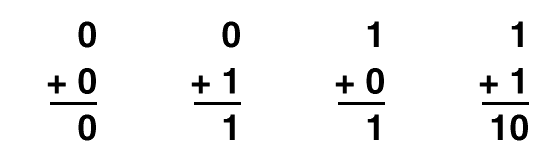Note that the decimal system 1 + 1 = 2 and in the binary system represent the decimal number 2 for binary number 10. The operation carried out, we realized the transport rule to the next column: 1 + 1 = 0 and carries 1 "will a".

The transport operation is also called carry, term derived from English.

Let's look at another example. Let's add up the binary numbers 11 and 10. The addition is made to column, column considering the transport from the previous column: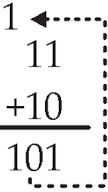1 + 1 = 0 and carries 1. Therefore, the sum of 11 + 10 binary results in 101.

5.2 � Subtraction in the binary system

The subtraction operation on binary numbers follows the same rule of subtracting decimal numbers.

Let's see the following example: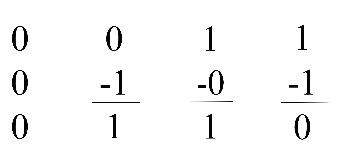Note that for operation 0-1 the result is equal to 1, but for the transport to the next column that must be accumulated in the subtrahend and, obviously, subtracted from the minuend.

To illustrate let's see, as an example, the operation of the following binary numbers: 111-100: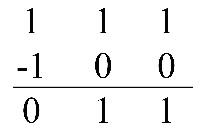Subtraction in binary numbers 111-100 = 11. In decimal, this operation is represented by 7-4 = 3.

To better understand the process of transporting the case 0-1, let's solve the binary operation step by step.

See: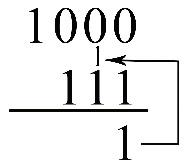0-1 = 1 and carries 1 to the next column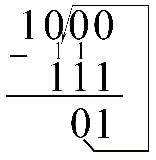0-1-1 = 0 and carries 1 to the next column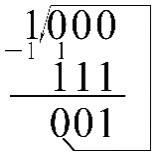0-1-1 = 0 and carries 1 to the next column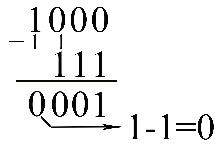1 � 1 = 0

So we have the result of this subtraction binary: 1000 � 111 = 0001

5.3 - Multiplication in binary system

Multiplication with binary numbers is performed the same way as in the decimal system.

In this way we have:

0 x 0 = 0

0 x 1 = 0

1 x 0 = 0

1 x 1 = 1

To illustrate, let's make the operation with binary numbers 11010 x 10: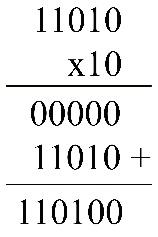The result of the multiplication of binary numbers 11010 x 10 is equal to 110100.

6 - Conclusion

This article has shown the basics of the main arithmetic operations with decimal numbers: binary, octal and hexadecimal. Operations are very important to the knowledge of those who study and/or work in the areas of Digital Electronics and computing.

One can check that the differences between transactions between the decimal numbers and decimal are not in its representation, because the logic and equality of results between the systems are perfectly visible.